Next: Equations for the free Up: Discreteness and its effect Previous: Discreteness and its effect

# Introduction

Normal state of the sea surface is chaotic with a lot of waves at different scales propagating in random directions. Such a state is referred to as Wave Turbulence (WT). Theory of WT was developed by finding a statistical closure based on the small nonlinearity and on the Wick splitting of the Fourier moments, the later procedure is often interpreted as closeness of statistics to Gaussian or/and to phase randomness (the two are not the same, see [6,4,5]). This closure yields a wave-kinetic equation (WKE) for the waveaction spectrum. Such WKE for the surface waves was first derived by Hasselmann . A significant achievement in WT theory was to realize that the most relevant states in WT are energy cascades through scales similar to the Kolmogorov cascades in Navier-Stokes turbulence, rather than thermodynamic equilibria as in the statistical theory of gases. This understanding came when Zakharov and Filonenko found an exact power-law solution to WKE which is similar to the famous Kolmogorov spectrum .

Numerical simulation of the moving water surface is a challenging problem due to a tremendous amount of computing power required for computing weakly nonlinear dispersive waves. This arises due to presence of widely separated spatial and time scales. As will be explained below, the weaker we take nonlinearity, the larger we should take the computational box in order to overcome the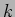-space discreteness and ignite wave resonances leading to the energy cascade through scales. In order to maximize the inertial range, one tries to force at the lowest wavenumbers possible, but without forgetting that the forcing should be strong enough for the resonance broadening to overcome the discreteness effect. But nonlinearity tends to grow along the energy cascade toward high's [9,10] and, therefore, the forcing at low wavenumbers should not be too strong for the nonlinearity to remain weak throughout the inertial range. A simple estimate  says that for the resonant interactions to be fully efficient, one must have a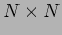computational box with the number of modes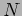related to the mean surface angle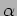as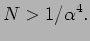Thus, for small enough nonlinearity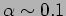one must have at least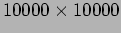resolution which is far beyond present computational capacity. Thus, in none of the existing numerical experiments, nor in near future, the formal applicability conditions of WT can be realized. On the other hand, a small fraction of the resonances can be activated at levels of nonlinearity which is much less than in the above estimate and this reduced set of resonant modes can, in principle, be sufficient to carry the turbulent cascade through scales. In this paper we estimate the minimal resonance broadening which is sufficient to generate the cascade.

It is necessary to examine which of the WT predictions survive beyond the formal applicability conditions when the cascade is carried by a depleted set of resonant modes, and which specific features arise due to such resonance depletion.

As in other recent numerical experiments [1,2,3], here we observe formation of a spectrum consistent with the ZF spectrum corresponding to the direct energy cascade. For this, the resonance broadening at the forcing scale should be maintained at about an order of magnitude larger than the minimal level necessary for triggering the cascade. Further, in agreement with more recent WT predictions about the higher-order statistics [4,5], we observe an anomalously high (with respect to Gaussian) probability of the large-amplitude waves. Also in agreement with recent WT findings , we observe a buildup of strong correlations of the wave phases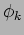whereas the factors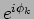remain de-correlated.

There are also distinct features arising due to discreteness. We analyze them by exploiting the two-peak structure of the time-Fourier transform at each: a dominant peak at (very close to) linear frequency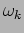, and a weaker one with a frequency approximately equal to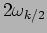. The second peak is a contribution of the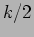-mode in the nonlinear term of the canonical transformation relating the normal variable and the observables (e.g. surface elevation). In fact, the nature of the second frequency peak is quite well understood in literature and it has even been used for remote sensing of vertical sheer by VHF frequency radars . In absence of nonlinearity one would observe only the first but not the second peak and, therefore, one can quantify the nonlinearity level as the ratio of the amplitudes of these two peaks. When the second peak becomes stronger than the first one, the wave phase experiences a rapid and persistent monotonic change. Detecting such phase runs'' gives an interesting picture of the nonlinear activity in the 2D-space. In particular, we notice a bursty'' nature of the energy cascade resembling sandpile avalanches. Possible explanation of such behavior is the following. When nonlinearity is weak, there is no wave-wave resonances, consequently there is no effective energy transfer, and system behaves like frozen turbulence'' (term introduced in  for the capillary wave turbulence). Energy generated at the forcing scale will accumulate near this scale and the nonlinearity will grow. When resonance broadening gets wide enough, so that the resonances are not inhibited by discreteness, the nonlinear wave-wave energy transfer starts, which diminishes nonlinearity and subsequently arrests'' resonances. Thus the system oscillates between having almost linear oscillations with stagnated energy and occasional avalanche-like discharges.Next: Equations for the free Up: Discreteness and its effect Previous: Discreteness and its effect
Dr Yuri V Lvov 2007-01-16